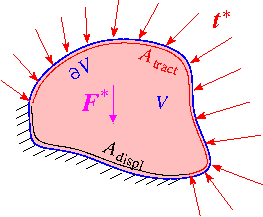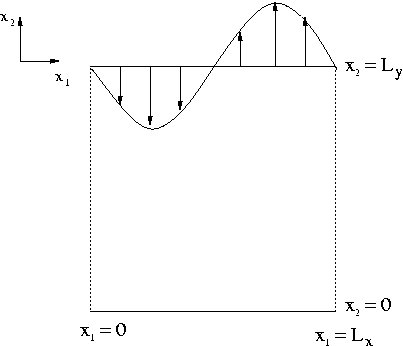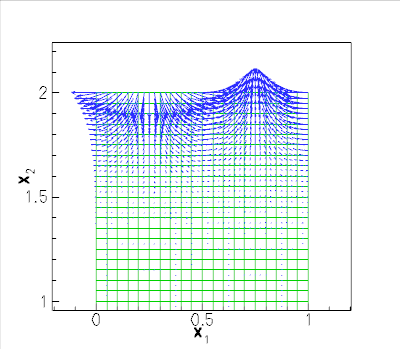Example problem: The deformation of an elastic strip by a periodic traction

This is our first linear elasticity example problem. We discuss the non-dimensionalisation of the governing equations and their implementation in oomph-lib, and then demonstrate the solution of a 2D problem: the deformation of an elastic strip by a periodic traction.

 Acknowledgement: This tutorial and the associated driver code was developed jointly with David Rutter.

# The governing equations

The figure below shows a sketch of a general elasticity problem. A linearly elastic solid body occupies the domainand is loaded by a body forceand by a surface tractionwhich is applied along part of its boundary,. The displacement is prescribed along the remainder of the boundary,, where.Sketch of a general elasticity problem: A linearly elastic body is loaded by a body force and is exposed to a prescribed traction along part of its boundary while the displacement is prescribed along the remainder of the boundary.

We adopt an Eulerian approach and describe the deformation in terms of the displacement fieldwhereandare the spatial coordinates and time, respectively. Throughout this document we will use index notation and the summation convention, and use asterisks to distinguish dimensional quantities from their non-dimensional counterparts.
Denoting the density of the body by, the deformation is governed by the Cauchy equations,whereis the Cauchy stress tensor which, for a linearly elastic solid, is given bywhereis the strain tensor,is the 4th order elasticity tensor, which for a homogeneous and isotropic solid iswhereis Young's modulus,is the Poisson ratio andis the Kronecker delta. Thus the Cauchy stress is given in terms of the displacement derivatives byWe non-dimensionalise the equations, using a problem specific reference length,, and a timescale, and use Young's modulus to non-dimensionalise the body force and the stress,The non-dimensional form of the Cauchy equations is then given bywhereThe parameteris the ratio of the elastic body's intrinsic timescale,, to the problem-specific timescale,, that we used to non-dimensionalise time.

The displacement constraints provide a Dirichlet condition for the displacements,while the traction boundary conditions require thatwhere theare the components of the outer unit normal to the boundary.

In this tutorial we only consider steady problems for which the equations reduce to# Implementation

## The elements

Within oomph-lib, the non-dimensional version of the DIM-dimensional Cauchy equations (1) with the constitutive equations (2) are implemented in the LinearElasticityEquations<DIM> equations class. Following our usual approach, discussed in the (Not-So-)Quick Guide, this equation class is then combined with a geometric finite element to form a fully-functional finite element. For instance, the combination of the LinearElasticityEquations<2> class with the geometric finite element QElement<2,3> yields a nine-node quadrilateral linear elasticity element. As usual, the mapping between local and global (Eulerian) coordinates within an element is given by,whereis the number of nodes in the element,is the-th global (Eulerian) coordinate of the-th Node in the element, and theare the element's shape functions, defined in the geometric finite element.

The cartesian displacement components[and] are stored as nodal values, and the shape functions are used to interpolate the displacements aswhereis the-th displacement component at the-th Node in the element. Nodal values of the displacement components are accessible via the access function

LinearElasticityEquations<DIM>::u(i,j)

which returns the-th displacement component stored at the element's-th Node.

# The example problem

To illustrate the solution of the steady equations of linear elasticity, we consider the 2D problem shown in the sketch below.

 The problem.Infinitely long strip loaded by a periodic traction. Solvein the domain, subject to the Dirichlet boundary conditionson the bottom boundary, the Neumann (traction) boundary conditionson the top boundary, and symmetry conditions atand,We note that forthe problem converges to the analytical solution.# Results

The figure below shows a vector plot of the displacement field near the upper domain boundary forand.
Note that we only discretised the infinite strip over one period of the applied, spatially-periodic surface traction, and imposed symmetry conditions on the left and right mesh boundaries.

The plot shows that the displacements decay rapidly with distance from the loaded surface – as suggested by the analytical solution for the infinite depth case. This suggests that the computation could greatly benefit from the use of spatial adaptivity. This is indeed the case and is explored in another tutorial.Plot of the displacement field.

# Global parameters and functions

As usual, we define all non-dimensional parameters in a namespace.

//===start_of_namespace=================================================
/// Namespace for global parameters
//======================================================================
{
/// Amplitude of traction applied
double Amplitude = 1.0;
/// Specify problem to be solved (boundary conditons for finite or
/// infinite domain).
bool Finite=false;
/// Define Poisson coefficient Nu
double Nu = 0.3;
/// Length of domain in x direction
double Lx = 1.0;
/// Length of domain in y direction
double Ly = 2.0;
/// The elasticity tensor
IsotropicElasticityTensor E(Nu);
/// The exact solution for infinite depth case
void exact_solution(const Vector<double> &x,
Vector<double> &u)
{
u = -Amplitude*cos(2.0*MathematicalConstants::Pi*x/Lx)*
exp(2.0*MathematicalConstants::Pi*(x-Ly))/
(2.0/(1.0+Nu)*MathematicalConstants::Pi);
u = -Amplitude*sin(2.0*MathematicalConstants::Pi*x/Lx)*
exp(2.0*MathematicalConstants::Pi*(x-Ly))/
(2.0/(1.0+Nu)*MathematicalConstants::Pi);
}
/// The traction function
void periodic_traction(const double &time,
const Vector<double> &x,
const Vector<double> &n,
Vector<double> &result)
{
result = -Amplitude*cos(2.0*MathematicalConstants::Pi*x/Lx);
result = -Amplitude*sin(2.0*MathematicalConstants::Pi*x/Lx);
}
} // end_of_namespace
Namespace for global parameters.
void periodic_traction(const double &time, const Vector< double > &x, const Vector< double > &n, Vector< double > &result)
The traction function.
double Amplitude
Amplitude of traction applied.
double Nu
Define Poisson coefficient Nu.
double Ly
Length of domain in y direction.
IsotropicElasticityTensor E(Nu)
The elasticity tensor.
bool Finite
Specify problem to be solved (boundary conditons for finite or infinite domain).
void exact_solution(const Vector< double > &x, Vector< double > &u)
The exact solution for infinite depth case.
double Lx
Length of domain in x direction.

# The driver code

We start by setting the number of elements in each of the two coordinate directions before creating a DocInfo object to store the output directory.

//===start_of_main======================================================
/// Driver code for PeriodicLoad linearly elastic problem
//======================================================================
int main(int argc, char* argv[])
{
// Number of elements in x-direction
unsigned nx=5;
// Number of elements in y-direction (for (approximately) square elements)
unsigned ny=unsigned(double(nx)*Global_Parameters::Ly/Global_Parameters::Lx);
// Set up doc info
DocInfo doc_info;
// Set output directory
doc_info.set_directory("RESLT");
int main(int argc, char *argv[])
Driver code for PeriodicLoad linearly elastic problem.

We build the problem using two-dimensional QLinearElasticityElements, solve using the Problem::newton_solve() function, and document the results.

// Set up problem
// Solve
problem.newton_solve();
// Output the solution
problem.doc_solution(doc_info);
} // end_of_main

# The problem class

The Problem class is very simple. As in other problems with Neumann boundary conditions, we provide separate meshes for the "bulk" elements and the face elements that apply the traction boundary conditions. The latter are attached to the relevant faces of the bulk elements by the function assign_traction_elements().

//===start_of_problem_class=============================================
//======================================================================
template<class ELEMENT>
{
public:
/// Constructor: Pass number of elements in x and y directions
/// and lengths
PeriodicLoadProblem(const unsigned &nx, const unsigned &ny,
const double &lx, const double &ly);
/// Update before solve is empty
/// Update after solve is empty
/// Doc the solution
void doc_solution(DocInfo& doc_info);
private:
/// Allocate traction elements on the top surface
/// Pointer to the bulk mesh
Mesh* Bulk_mesh_pt;
/// Pointer to the mesh of traction elements
}; // end_of_problem_class
Mesh * Bulk_mesh_pt
Pointer to the bulk mesh.
Mesh * Surface_mesh_pt
Pointer to the mesh of traction elements.
PeriodicLoadProblem(const unsigned &nx, const unsigned &ny, const double &lx, const double &ly)
Constructor: Pass number of elements in x and y directions and lengths.
void actions_before_newton_solve()
Update before solve is empty.
void actions_after_newton_solve()
Update after solve is empty.
void doc_solution(DocInfo &doc_info)
Doc the solution.
void assign_traction_elements()
Allocate traction elements on the top surface.

# The problem constructor

Since this is a steady problem, the constructor is quite simple. We begin by building the meshes and pin the displacements on the appropriate boundaries. We then assign the boundary values for the displacements along the bottom boundary. We either set the displacements to zero or assign their values from the exact solution for the infinite depth case.

//===start_of_constructor=============================================
/// Problem constructor: Pass number of elements in coordinate
/// directions and size of domain.
//====================================================================
template<class ELEMENT>
(const unsigned &nx, const unsigned &ny,
const double &lx, const double& ly)
{
//Now create the mesh with periodic boundary conditions in x direction
bool periodic_in_x=true;
Bulk_mesh_pt =
//Create the surface mesh of traction elements
Surface_mesh_pt=new Mesh;
assign_traction_elements();
// Set the boundary conditions for this problem: All nodes are
// free by default -- just pin & set the ones that have Dirichlet
// conditions here
unsigned ibound=0;
unsigned num_nod=Bulk_mesh_pt->nboundary_node(ibound);
for (unsigned inod=0;inod<num_nod;inod++)
{
// Get pointer to node
Node* nod_pt=Bulk_mesh_pt->boundary_node_pt(ibound,inod);
// Pinned in x & y at the bottom and set value
nod_pt->pin(0);
nod_pt->pin(1);
// Check which boundary conditions to set and set them
{
// Set the displacements to zero
nod_pt->set_value(0,0);
nod_pt->set_value(1,0);
}
else
{
// Extract nodal coordinates from node:
Vector<double> x(2);
x=nod_pt->x(0);
x=nod_pt->x(1);
// Compute the value of the exact solution at the nodal point
Vector<double> u(2);
// Assign these values to the nodal values at this node
nod_pt->set_value(0,u);
nod_pt->set_value(1,u);
};
} // end_loop_over_boundary_nodes

Next we pass a pointer to the elasticity tensor (stored in Global_Physical_Variables::E) to all elements.

// Complete the problem setup to make the elements fully functional
// Loop over the elements
unsigned n_el = Bulk_mesh_pt->nelement();
for(unsigned e=0;e<n_el;e++)
{
// Cast to a bulk element
ELEMENT *el_pt = dynamic_cast<ELEMENT*>(Bulk_mesh_pt->element_pt(e));
// Set the elasticity tensor
el_pt->elasticity_tensor_pt() = &Global_Parameters::E;
}// end loop over elements

We loop over the traction elements and specify the applied traction.

// Loop over the traction elements
unsigned n_traction = Surface_mesh_pt->nelement();
for(unsigned e=0;e<n_traction;e++)
{
// Cast to a surface element
LinearElasticityTractionElement<ELEMENT> *el_pt =
dynamic_cast<LinearElasticityTractionElement<ELEMENT>* >
(Surface_mesh_pt->element_pt(e));
// Set the applied traction
el_pt->traction_fct_pt() = &Global_Parameters::periodic_traction;
}// end loop over traction elements

The two submeshes are now added to the problem and a global mesh is constructed before the equation numbering scheme is set up, using the function assign_eqn_numbers().

// Add the submeshes to the problem
// Now build the global mesh
build_global_mesh();
// Assign equation numbers
cout << assign_eqn_numbers() << " equations assigned" << std::endl;
} // end of constructor

# The traction elements

In anticipation of the extension of this code to its adaptive counterpart, we create the face elements that apply the traction to the upper boundary in a separate function.

//===start_of_traction===============================================
/// Make traction elements along the top boundary of the bulk mesh
//===================================================================
template<class ELEMENT>
{
// How many bulk elements are next to boundary 2 (the top boundary)?
unsigned bound=2;
unsigned n_neigh = Bulk_mesh_pt->nboundary_element(bound);
// Now loop over bulk elements and create the face elements
for(unsigned n=0;n<n_neigh;n++)
{
// Create the face element
FiniteElement *traction_element_pt
= new LinearElasticityTractionElement<ELEMENT>
(Bulk_mesh_pt->boundary_element_pt(bound,n),
Bulk_mesh_pt->face_index_at_boundary(bound,n));
}
} // end of assign_traction_elements

# Post-processing

As expected, this member function documents the computed solution.

//==start_of_doc_solution=================================================
/// Doc the solution
//========================================================================
template<class ELEMENT>
{
ofstream some_file;
char filename;
// Number of plot points
unsigned npts=5;
// Output solution
sprintf(filename,"%s/soln.dat",doc_info.directory().c_str());
some_file.open(filename);
Bulk_mesh_pt->output(some_file,npts);
some_file.close();
// Output exact solution
sprintf(filename,"%s/exact_soln.dat",doc_info.directory().c_str());
some_file.open(filename);
Bulk_mesh_pt->output_fct(some_file,npts,
some_file.close();
// Doc error
double error=0.0;
double norm=0.0;
sprintf(filename,"%s/error.dat",doc_info.directory().c_str());
some_file.open(filename);
Bulk_mesh_pt->compute_error(some_file,
error,norm);
some_file.close();
// Doc error norm:
cout << "\nNorm of error " << sqrt(error) << std::endl;
cout << "Norm of solution : " << sqrt(norm) << std::endl << std::endl;
cout << std::endl;
} // end_of_doc_solution

As discussed in the introduction, the non-dimensional version of the steady Cauchy equations only contains a single non-dimensional parameter, the Poisson ratiowhich is passed to the constructor of the IsotropicElasticityTensor. If you inspect the relevant source code src/linear_elasticity/elasticity_tensor.h you will find that this constructor has a second argument which defaults to one. This argument plays the role of Young's modulus and is best interpreted as the ratio of the material's actual Young's modulus to the (nominal) Young's modulus used in the non-dimensionalisation of the equations. The ability to provide this ratio is important if different regions of the body contain materials with different material properties.

## Exercises

1. Fix the size of the domain and set the displacements along the bottom boundary to the exact solution for the infinite depth case, i.e., using the Global_Parameters::Finite flag. Then investigate how the solution converges to the exact solution for increasing numbers of elements.
2. Try varying the depth of the domain by changing Global_Parameters::Ly while maintaining a constant spatial resolution (i.e. increasing the number of elements – this is already done in the driver code where we compute ny in terms of Global_Parameters::Ly ) and compare how the solution converges to the exact solution of the infinite depth case.